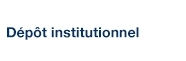# Codings of rotations on two intervals are full

Blondin Massé, Alexandre; Brlek, Srecko; Labbé, Sébastien et Vuillon, Laurent (2009). « Codings of rotations on two intervals are full ». Electronic Notes in Discrete Mathematics, 34, pp. 289-293.

Fichier(s) associé(s) à ce document :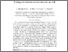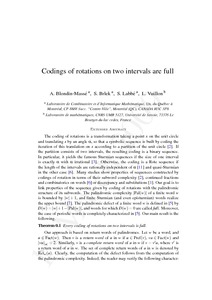Prévisualisation
PDF
Télécharger (61kB)

## Résumé

Codings of rotations are transformations taking a point x on the unit circle and translating it by an angle α, so that a symbolic sequence is built by coding iterations of this translation on x according to a partition of the unit circle [P. Alessandri and V. Berthé, Three distance theorems and combinatorics on words, Enseig. Math. 44 (1998) 103–132]. If the partition consists of two intervals, the resulting coding is a binary sequence. In particular, it yields the famous Sturmian sequences if the size of one interval is exactly α with α irrational [J. Berstel and P. Séébold, Sturmian words, in: Lothaire, Combinatorics on Words, Cambridge University Press, 2002]. Otherwise, the coding is a Rote sequence if the length of the intervals are rationally independent of α [G. Rote, Sequences with subword complexity 2n, J. Number Theory46 (1994) 196–213] and quasi-Sturmian in the other case [G. Didier, Codages de rotations et fractions continues, J. Number Theory 71 (1998) 275–306]. Many studies show properties of sequences constructed by codings of rotation in terms of their subword complexity [P. Alessandri and V. Berthé, Three distance theorems and combinatorics on words, Enseig. Math. 44 (1998) 103–132], continued fractions and combinatorics on words [G. Didier, Codages de rotations et fractions continues, J. Number Theory 71 (1998) 275–306] or discrepancy and substitutions [B. Adamczewski, Codages de rotations et phénomènes d'autosimilarité, J. Théor. nombres Bordeaux14 (2002) 351–386]. Our goal is to link properties of the sequence given by coding of rotations with the palindromic structure of its subwords. The palindromic complexity |Pal(w)||Pal(w)| of a finite word w is bounded by |w|+1|w|+1, and finite Sturmian (and even episturmian) words realize the upper bound [X. Droubay, J. Justin and G. Pirillo, Episturmian words and some constructions of de Luca and Rauzy, Theoret. Comput. Sci. 255 (2001) 539–553]. The palindromic defect of a finite word w is defined in [S. Brlek, S. Hamel, M. Nivat and C. Reutenauer, On the palindromic complexity of infinite words, Int. J. Found. Comput. Sci. 15: 2 (2004) 293–306] by D(w)=|w|+1−|Pal(w)|D(w)=|w|+1−|Pal(w)|. Words for which D(w)=0D(w)=0 are called full [S. Brlek, S. Hamel, M. Nivat and C. Reutenauer, On the palindromic complexity of infinite words, Int. J. Found. Comput. Sci. 15: 2 (2004) 293–306] or rich [A. Glen, J. Justin, S. Widmer and L.Q. Zamboni, Palindromic richness, Eur. J. Comb. 30 (2009) 510–531] and an infinite word is full if all its prefixes are full. Moreover, the case of periodic words is completely characterized in [S. Brlek, S. Hamel, M. Nivat and C. Reutenauer, On the palindromic complexity of infinite words, Int. J. Found. Comput. Sci. 15: 2 (2004) 293–306]. Our main result is the following.

Type: Article de revue scientifique Codings of rotations, Sturmian sequences, finite word, palindromic defect Faculté des sciences > Département d'informatique Alexandre Blondin Massé 10 mai 2016 13:10 30 mai 2016 14:46 http://archipel.uqam.ca/id/eprint/8433

## Statistiques

Voir les statistiques sur cinq ans...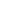RECHERCHER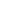PARCOURIR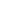LIBRE ACCÈS